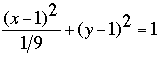SEARCH HOMEMath Central Quandaries & QueriesQuestion from Rebecca, a student: I have to write the following equation into standard form of an ellipse: 9(X-1)^2 + (Y+1)^2 = 1 As you know, standard form is in the following: (X)^2/a^2 + (Y)^2/b^2 = 1 If I decide both sides by nine then the one becomes 1/9. What do I do? Please get back to me ASAP. I need to do the math corrections by tomorrow morning. Thank you!We have two responses for you

Rebecca,Harley

Hi Rebecca.

An ellipse that isn't centered at the origin has the form

(x-h)2/a2 + (y-k)2/b2 = 1

Where (h, k) is the center of the ellipse.

Cheers,
Stephen La Rocque.Math Central is supported by the University of Regina and The Pacific Institute for the Mathematical Sciences.